# On the Unique Games Conjecture Subhash Khot NYU

• Slides: 52
Download presentation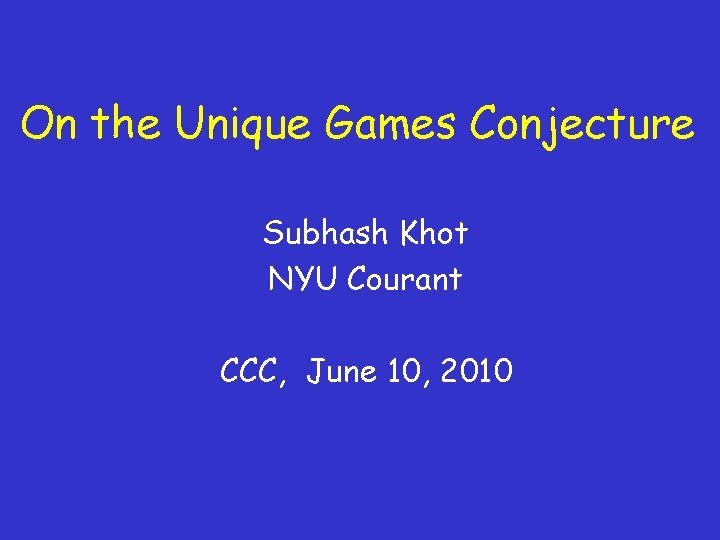On the Unique Games Conjecture Subhash Khot NYU Courant CCC, June 10, 2010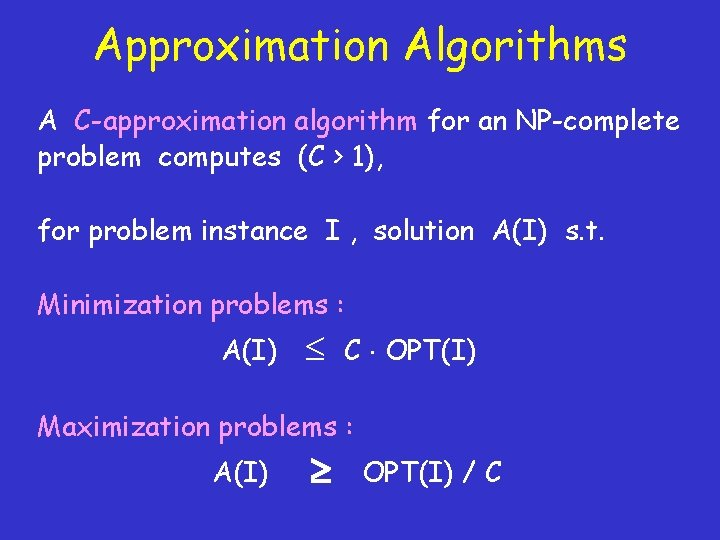Approximation Algorithms A C-approximation algorithm for an NP-complete problem computes (C > 1), for problem instance I , solution A(I) s. t. Minimization problems : A(I) C OPT(I) Maximization problems : A(I) OPT(I) / CPCP Theorem [B’ 85, GMR’ 89, BFL’ 91, LFKN’ 92, S’ 92, ……] [FGLSS’ 91, AS’ 92 ALMSS’ 92] [PY’ 91] Theorem : It is NP-hard to tell whether a MAX-3 SAT instance is * Satisfiable (i. e. OPT = 1) or * No assignment satisfies more than 99% clauses (i. e. OPT 0. 99). i. e. MAX-3 SAT is 1. 01 hard to approximate.(In)approximability : Towards Tight Hardness Results • [Hastad’ 96] • [Hastad’ 97] • [Feige’ 98] [Dinur’ 05] Clique n 1 - MAX-3 SAT 8/7 - Set Cover (1 - ) ln n Combinatorial Proof of PCP Theorem !Open Problems in (In) Approximability – Vertex Cover (1. 36 vs. 2) [Dinur. Safra’ 02] – Coloring 3 -colorable graphs (5 vs. n 3/14) [Khanna. Linial. Safra’ 93, Blum. Karger’ 97] – Sparsest Cut (1+ε vs. (logn)1/2) [AMS’ 07, Arora. Rao. Vazirani’ 04] – Max Cut (17/16 vs 1/0. 878… ) [Håstad’ 97, Goemans. Williamson’ 94] ……………. .Unique Games Conjecture [K’ 02] Supporting Evidence Connections to: • Inapproximability (UGC several problems inapproximable) • Discrete Fourier Analysis • Geometry • Algorithms (e. g. KKL, Majority is Stablest) (Isoperimetry, Metric geometry, Integrality gaps) (Attempts to disprove UGC) • Parallel Repetition (Gap amplification, Foam construction)Example of Unique Game (2 CSP) OPT = max fraction of equations that can be satisfied by any assignment. x 1 x 5 x 2 - x 3 = 2 (mod k) x 2 = -1 (mod k) x 1 = k-7 (mod k) ………….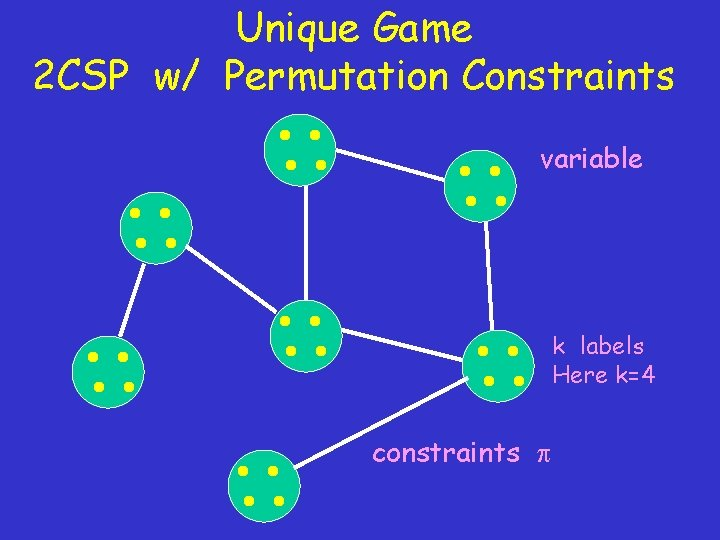Unique Game 2 CSP w/ Permutation Constraints variable k labels Here k=4 constraints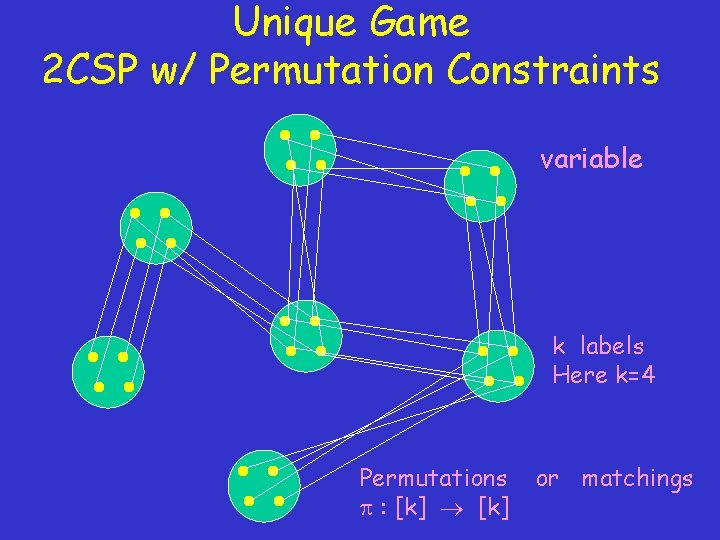Unique Game 2 CSP w/ Permutation Constraints variable k labels Here k=4 Permutations : [k] or matchings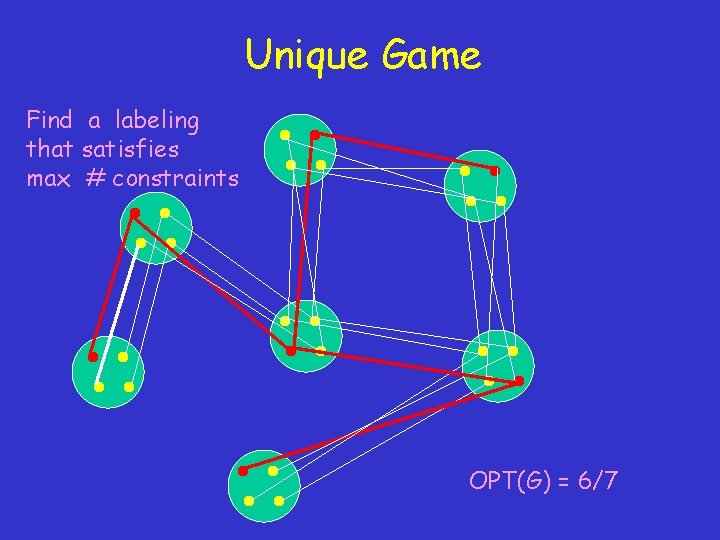Unique Game Find a labeling that satisfies max # constraints OPT(G) = 6/7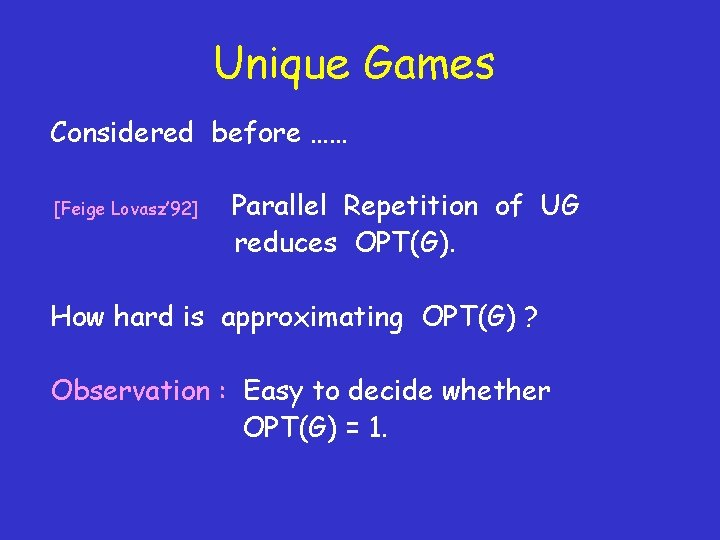Unique Games Considered before …… [Feige Lovasz’ 92] Parallel Repetition of UG reduces OPT(G). How hard is approximating OPT(G) ? Observation : Easy to decide whether OPT(G) = 1.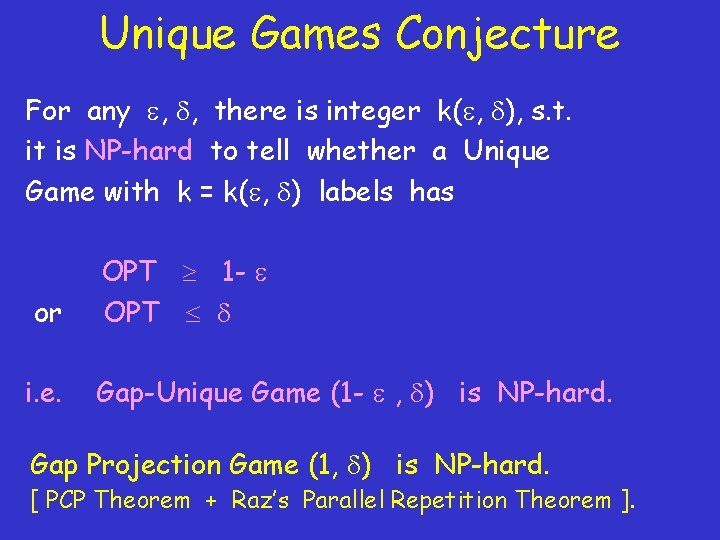Unique Games Conjecture For any , , there is integer k( , ), s. t. it is NP-hard to tell whether a Unique Game with k = k( , ) labels has or OPT 1 - OPT i. e. Gap-Unique Game (1 - , ) is NP-hard. Gap Projection Game (1, ) is NP-hard. [ PCP Theorem + Raz’s Parallel Repetition Theorem ].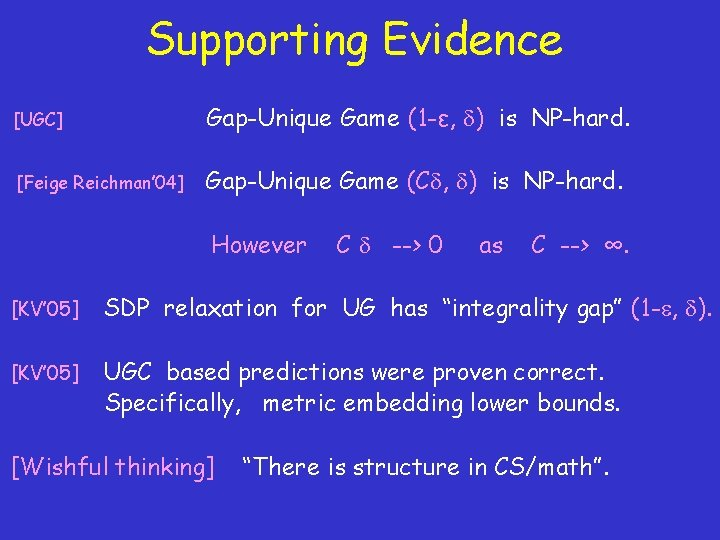Supporting Evidence [UGC] Gap-Unique Game (1 -ε, ) is NP-hard. [Feige Reichman’ 04] Gap-Unique Game (C , ) is NP-hard. However C --> 0 as C --> ∞. [KV’ 05] SDP relaxation for UG has “integrality gap” (1 - , ). [KV’ 05] UGC based predictions were proven correct. Specifically, metric embedding lower bounds. [Wishful thinking] “There is structure in CS/math”.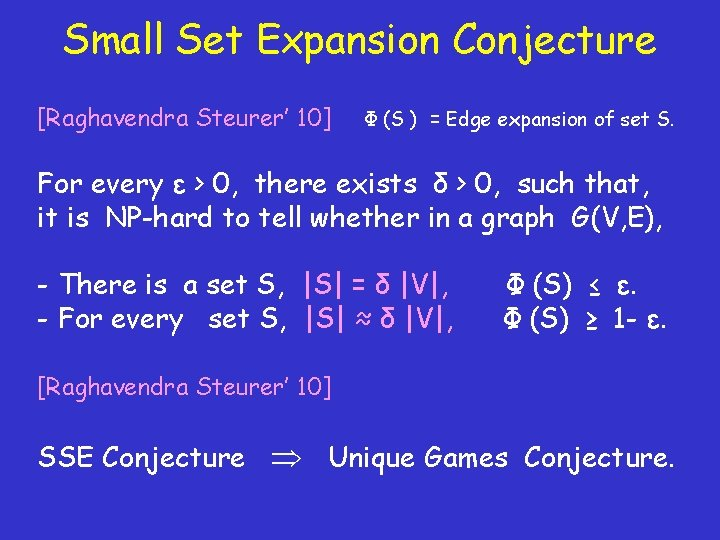Small Set Expansion Conjecture [Raghavendra Steurer’ 10] Φ (S ) = Edge expansion of set S. For every ε > 0, there exists δ > 0, such that, it is NP-hard to tell whether in a graph G(V, E), - There is a set S, |S| = δ |V|, - For every set S, |S| ≈ δ |V|, Φ (S) ≤ ε. Φ (S) ≥ 1 - ε. [Raghavendra Steurer’ 10] SSE Conjecture Unique Games Conjecture.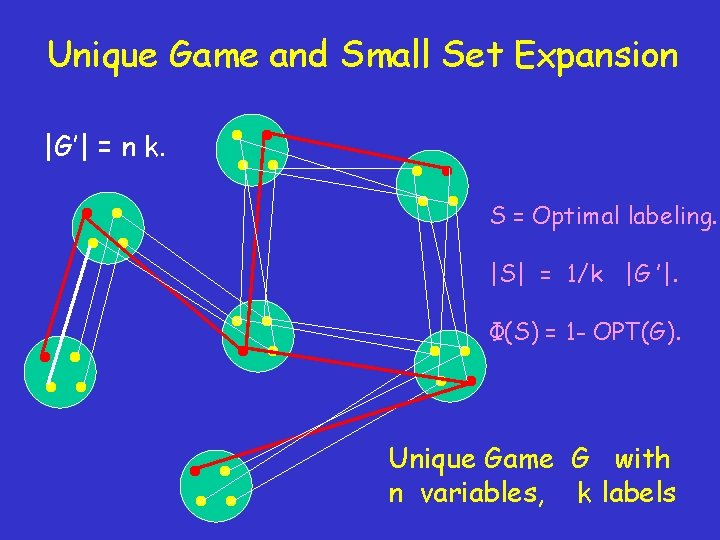Unique Game and Small Set Expansion |G’| = n k. S = Optimal labeling. |S| = 1/k |G ’|. Φ(S) = 1 - OPT(G). Unique Game G with n variables, k labels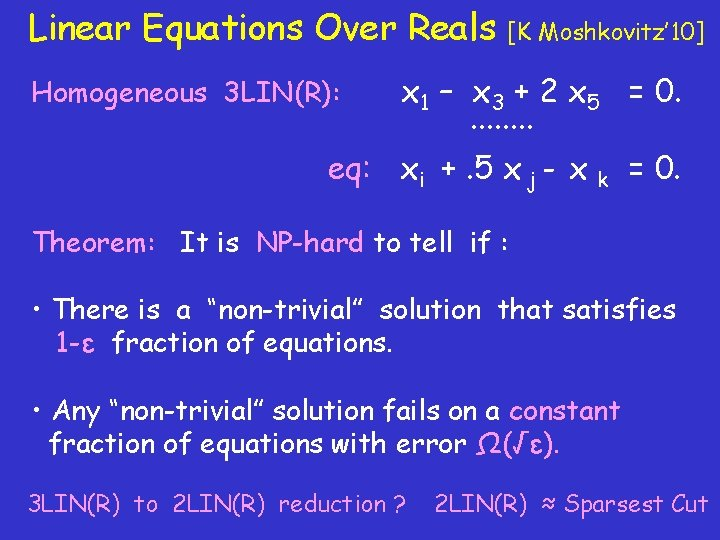Linear Equations Over Reals [K Moshkovitz’ 10] x 1 – x 3 + 2 x 5 = 0. ∙∙∙∙ eq: xi +. 5 x j - x k = 0. Homogeneous 3 LIN(R): Theorem: It is NP-hard to tell if : • There is a “non-trivial” solution that satisfies 1 -ε fraction of equations. • Any “non-trivial” solution fails on a constant fraction of equations with error Ω(√ε). 3 LIN(R) to 2 LIN(R) reduction ? 2 LIN(R) ≈ Sparsest Cut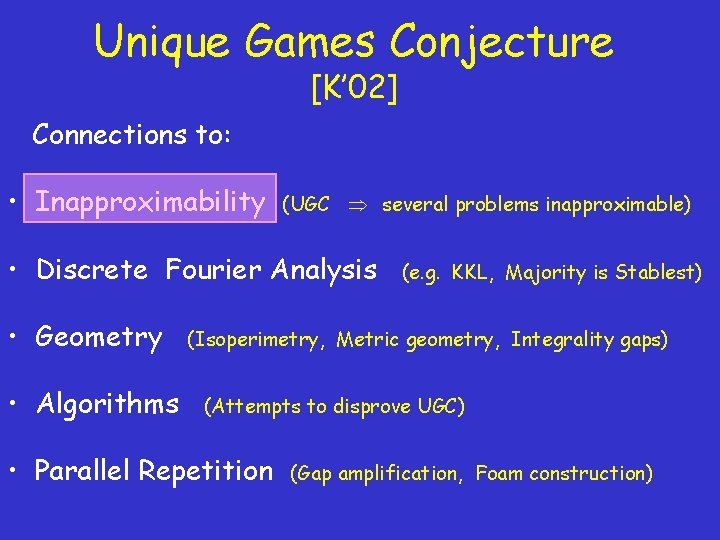Unique Games Conjecture [K’ 02] Connections to: • Inapproximability (UGC several problems inapproximable) • Discrete Fourier Analysis • Geometry • Algorithms (e. g. KKL, Majority is Stablest) (Isoperimetry, Metric geometry, Integrality gaps) (Attempts to disprove UGC) • Parallel Repetition (Gap amplification, Foam construction)Generic Reduction from Unique Game [BGS’ 95 (Long Code), Hastad’ 97 (Fourier), UGC , ……]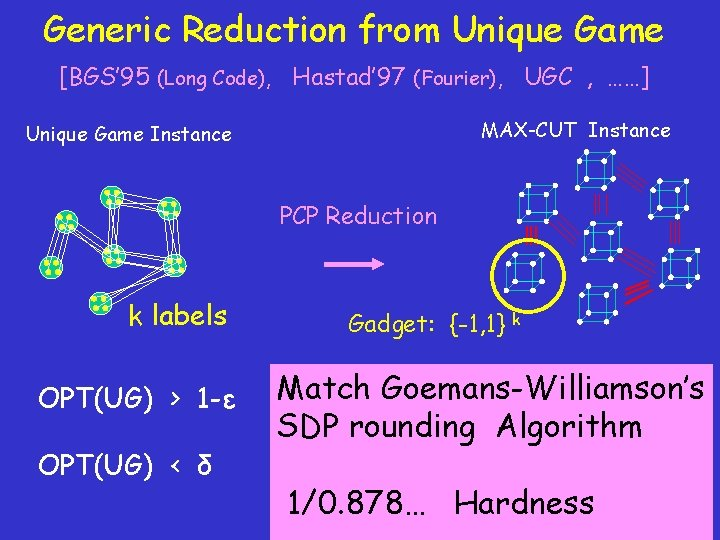Generic Reduction from Unique Game [BGS’ 95 (Long Code), Hastad’ 97 (Fourier), UGC , ……] MAX-CUT Instance Unique Game Instance PCP Reduction k labels OPT(UG) > 1 -ε OPT(UG) < δ Gadget: {-1, 1} k Match Goemans-Williamson’s sized cut. SDP rounding Algorithm No cut with size arccos (1 -2 ) / 1/0. 878… Hardness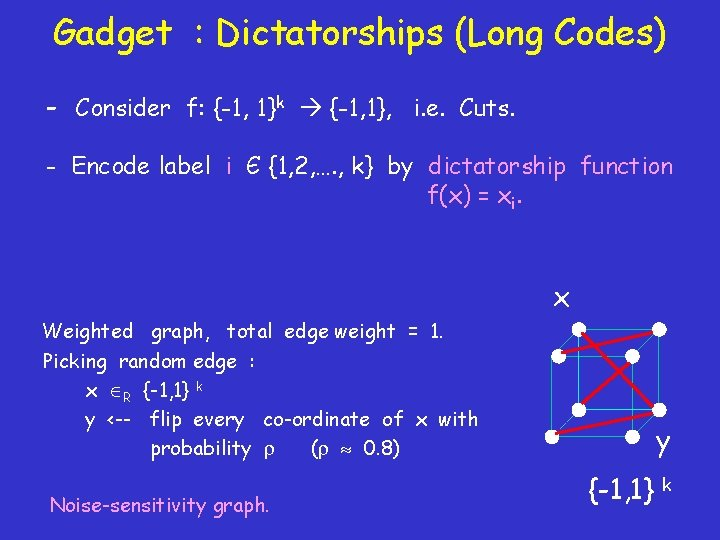Gadget : Dictatorships (Long Codes) - Consider f: {-1, 1}k {-1, 1}, i. e. Cuts. - Encode label i Є {1, 2, …. , k} by dictatorship function f(x) = xi. x Weighted graph, total edge weight = 1. Picking random edge : x R {-1, 1} k y <-- flip every co-ordinate of x with probability ( 0. 8) Noise-sensitivity graph. y {-1, 1} k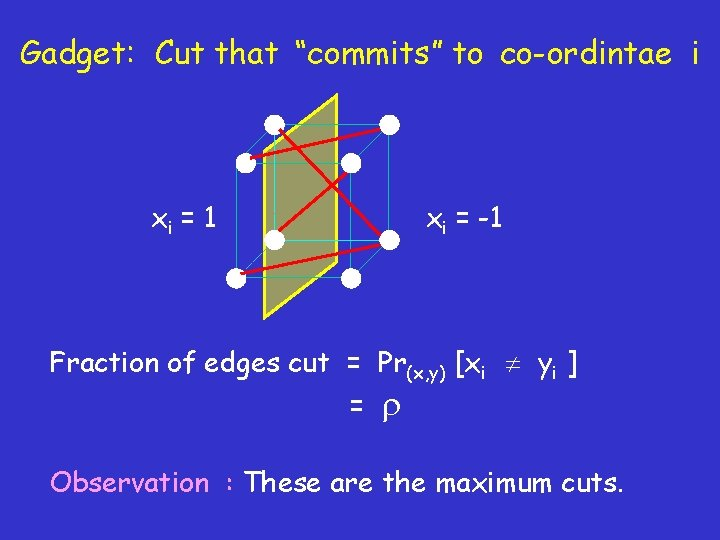Gadget: Cut that “commits” to co-ordintae i xi = 1 xi = -1 Fraction of edges cut = Pr(x, y) [xi yi ] = Observation : These are the maximum cuts.Gadget : Cuts not committing to a co-ordinate How large can be cuts with no influential co-ordinate ? Influence (i, f) = Pr [ f(x) f(x+e ) ] x Random Cut : ½ Majority Cut : > arccos (1 -2 ) / > ½ [KKMO’ 04, MOO’ 05] Majority Is Stablest (Under Noise) Any cut with no influential co-ordinate has size at most arccos (1 -2 ) / . i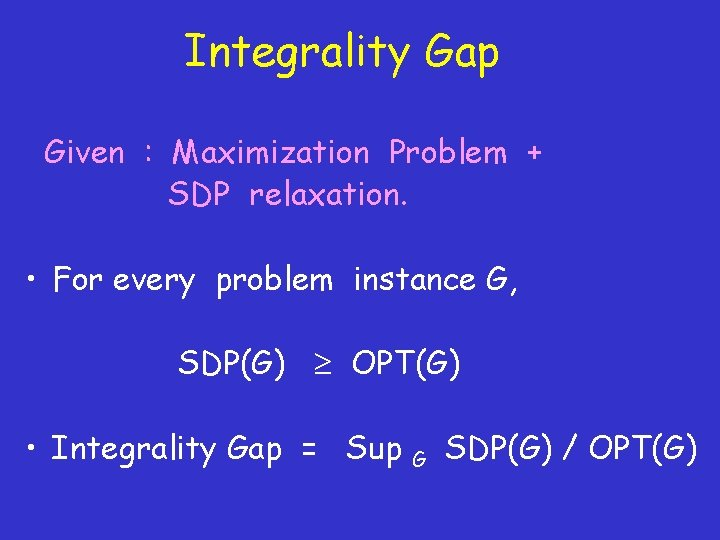Integrality Gap Given : Maximization Problem + SDP relaxation. • For every problem instance G, SDP(G) OPT(G) • Integrality Gap = Sup G SDP(G) / OPT(G)[Raghavendra’ 08] • Duality between Algorithms and Hardness. • For every CSP, write a natural SDP relaxation. • Integrality gap = β. Implies β-approximation. • Theorem: Every instance with gap β’ < β can be used to construct a gadget and prove UGC-based β’- hardness result ! • SDPs are optimal algorithm for CSPs.Unique Games Conjecture [K’ 02] Connections to: • Inapproximability (UGC several problems inapproximable) • Discrete Fourier Analysis • Geometry • Algorithms (e. g. KKL, Majority is Stablest) (Isoperimetry, Metric geometry, Integrality gaps) (Attempts to disprove UGC) • Parallel Repetition (Gap amplification, Foam construction)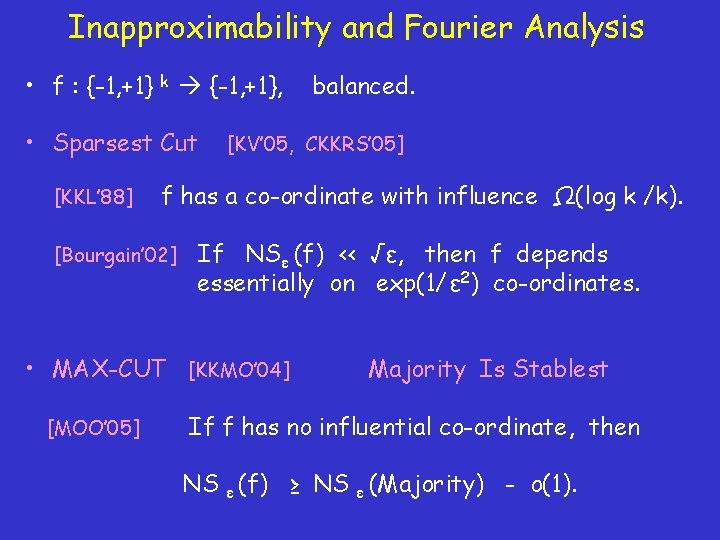Inapproximability and Fourier Analysis • f : {-1, +1} k {-1, +1}, • Sparsest Cut [KKL’ 88] [KV’ 05, CKKRS’ 05] f has a co-ordinate with influence Ω(log k /k). [Bourgain’ 02] If NSε (f) << √ε, then f depends essentially on exp(1/ε 2) co-ordinates. • MAX-CUT [KKMO’ 04] [MOO’ 05] balanced. Majority Is Stablest If f has no influential co-ordinate, then NS ε (f) ≥ NS ε (Majority) - o(1).Inapproximability and Fourier • f : {-1, +1}k {-1, +1}, • Vertex Cover [Friedgut’ 98] [MOO’ 05] balanced. [Dinur. Safra’ 02, K Regev’ 03, K Bansal’ 09] If total influence is k, then f depends essentially on exp(k) co-ordintaes. It Ain’t Over Till It’s Over If f has no influential co-ordinate, then on almost every subcube of {-1, +1}k of dimension k/100, f = 1 and f = -1 with constant probability.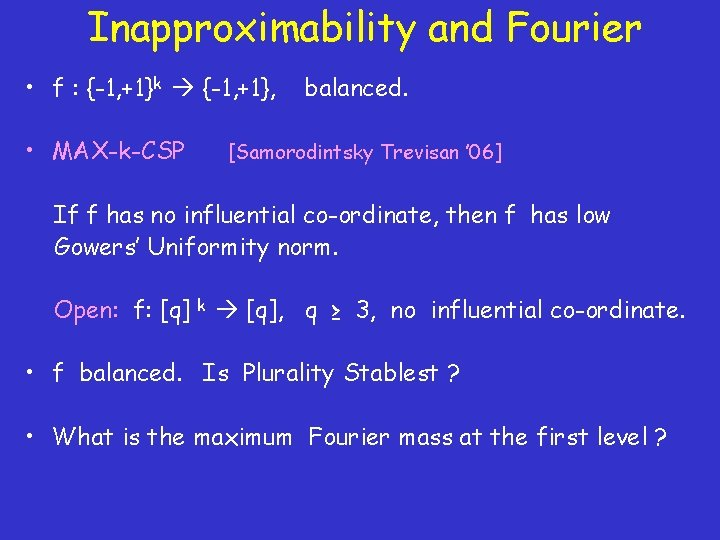Inapproximability and Fourier • f : {-1, +1}k {-1, +1}, • MAX-k-CSP balanced. [Samorodintsky Trevisan ’ 06] If f has no influential co-ordinate, then f has low Gowers’ Uniformity norm. Open: f: [q] k [q], q ≥ 3, no influential co-ordinate. • f balanced. Is Plurality Stablest ? • What is the maximum Fourier mass at the first level ?Unique Games Conjecture [K’ 02] Connections to: • Inapproximability (UGC several problems inapproximable) • Discrete Fourier Analysis • Geometry • Algorithms (e. g. KKL, Majority is Stablest) (Isoperimetry, Metric geometry, Integrality gaps) (Attempts to disprove UGC) • Parallel Repetition (Gap amplification, Foam construction)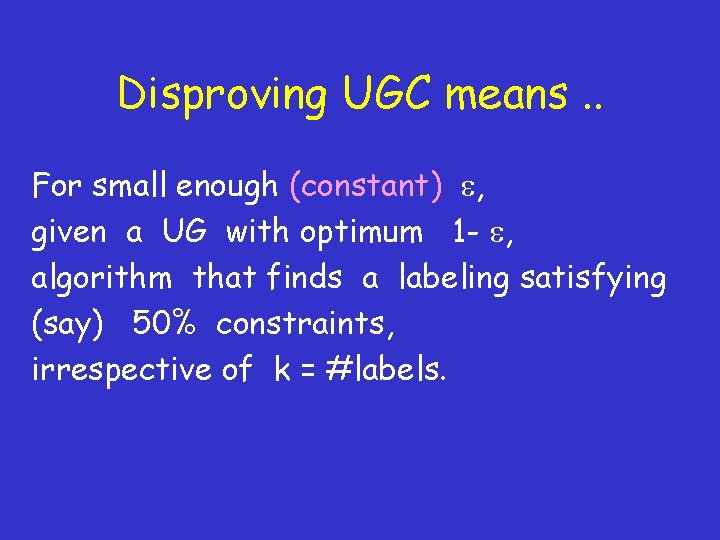Disproving UGC means. . For small enough (constant) , given a UG with optimum 1 - , algorithm that finds a labeling satisfying (say) 50% constraints, irrespective of k = #labels.Algorithmic Results Algorithm that finds a labeling satisfying f( , k, n) fraction of constraints. [K’ 02] [Trevisan’ 05] [Gupta Talwar’ 05] [CMM’ 06] 1 - 1/5 k 2 1 - 1/3 log 1/3 n 1 - log n 1/k , 1 - 1/2 log 1/2 k 1 - log 1/2 k log 1/2 n [AKKSTV’ 08 , Kolla’ 10] [ABS’ 10] UG on “mild” expander graphs. Exp ( n ) time algorithm. None of these disproves UGC. However …If the UGC is true, then : • k >> 21/ε. • Graph of constraints cannot even be a “mild” expander. UG is easy on random graphs. • Reduction from 3 SAT must blow up the size by n 1/ε. • Conjecture does not hold for sub-constant ε, i. e. below 1/log n.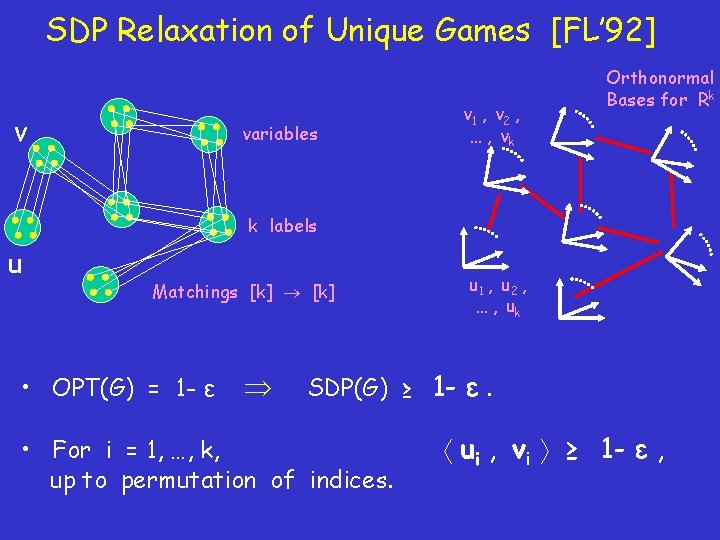SDP Relaxation of Unique Games [FL’ 92] v variables v 1 , v 2 , … , vk Orthonormal Bases for Rk k labels u Matchings [k] • OPT(G) = 1 - ε u 1 , u 2 , … , uk SDP(G) ≥ 1 - ε. • For i = 1, …, k, up to permutation of indices. ui , vi ≥ 1 - ε ,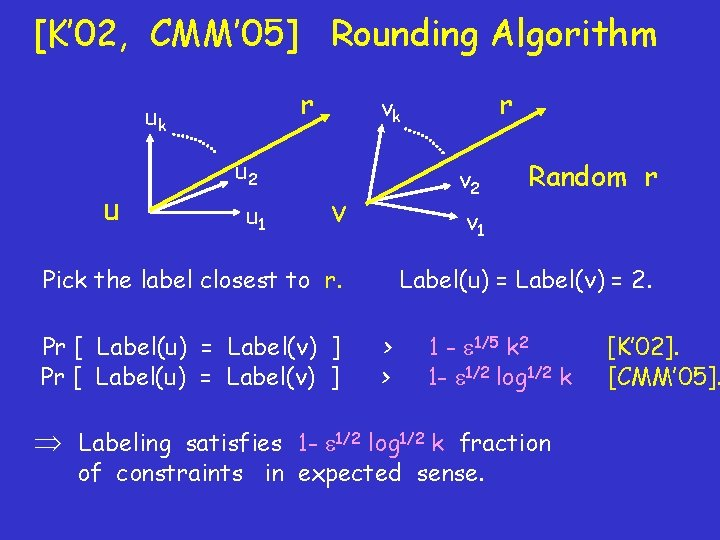[K’ 02, CMM’ 05] Rounding Algorithm r uk u u 2 u 1 r vk v 2 v v 1 Pick the label closest to r. Pr [ Label(u) = Label(v) ] Random r Label(u) = Label(v) = 2. > > 1 - 1/5 k 2 1 - 1/2 log 1/2 k Labeling satisfies 1 - 1/2 log 1/2 k fraction of constraints in expected sense. [K’ 02]. [CMM’ 05].[Trevisan’ 05] Algorithm Graph of variables and constraints • [Leighton Rao’ 88] Delete 1% of edges so that all connected components have diameter O(log n). • Algorithm to solve UG on low diameter graph.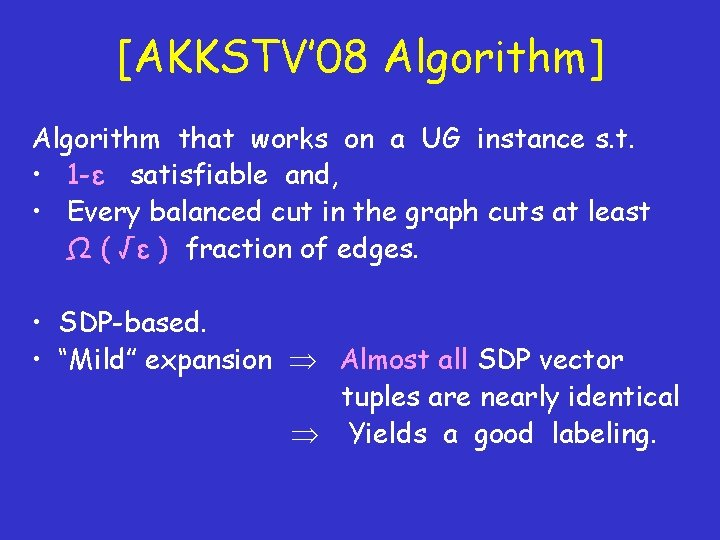[AKKSTV’ 08 Algorithm] Algorithm that works on a UG instance s. t. • 1 -ε satisfiable and, • Every balanced cut in the graph cuts at least Ω ( √ε ) fraction of edges. • SDP-based. • “Mild” expansion Almost all SDP vector tuples are nearly identical Yields a good labeling.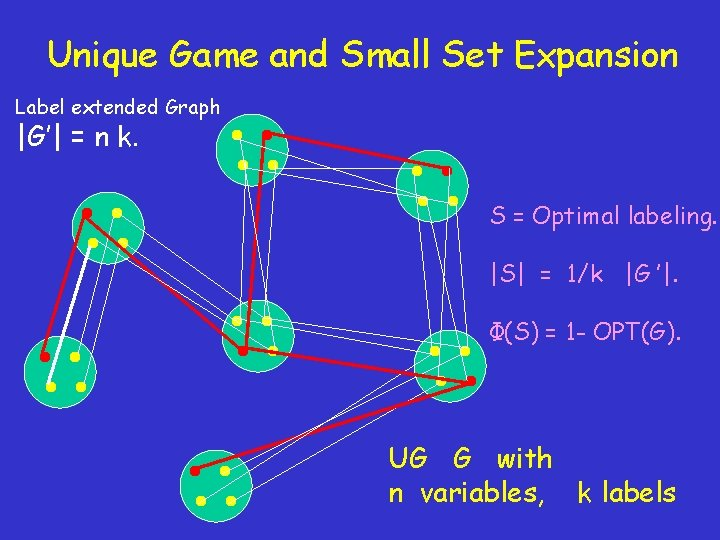Unique Game and Small Set Expansion Label extended Graph |G’| = n k. S = Optimal labeling. |S| = 1/k |G ’|. Φ(S) = 1 - OPT(G). UG G with n variables, k labels[Arora Barak Steurer’ 10 Algorithm] [Kolla’ 10, Naor’ 10] • Algo. runs in time exp(nε ) on UG that is 1 -ε satisfiable. • Good solution to UG Small non-expanding set S in G’. • Small non-expanding set in label-extended graph G’ Either corresponds to a good UG solution Or is a non-expanding set in G (useful) (fake). • Iteratively remove all fake sets from G, sacrificing at most 1% edges.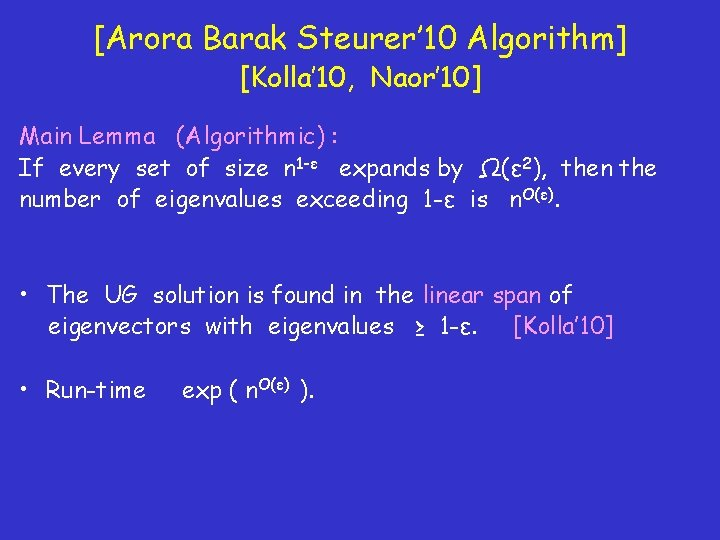[Arora Barak Steurer’ 10 Algorithm] [Kolla’ 10, Naor’ 10] Main Lemma (Algorithmic) : If every set of size n 1 -ε expands by Ω(ε 2), then the number of eigenvalues exceeding 1 -ε is n. O(ε). • The UG solution is found in the linear span of eigenvectors with eigenvalues ≥ 1 -ε. [Kolla’ 10] • Run-time exp ( n. O(ε) ).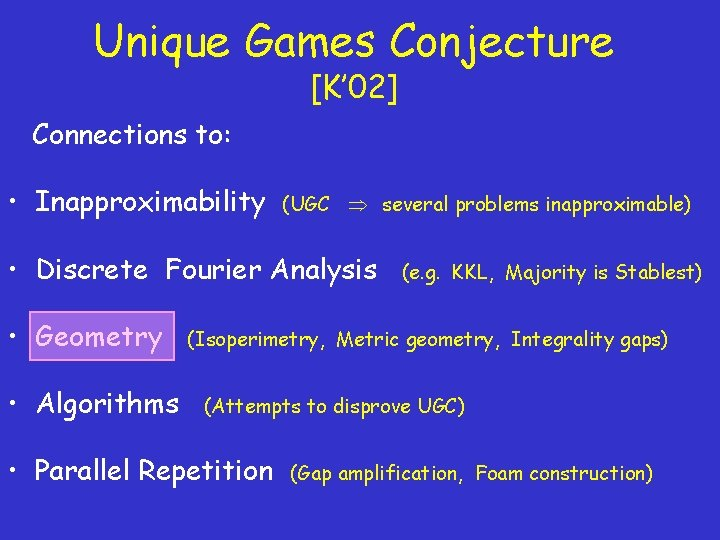Unique Games Conjecture [K’ 02] Connections to: • Inapproximability (UGC several problems inapproximable) • Discrete Fourier Analysis • Geometry • Algorithms (e. g. KKL, Majority is Stablest) (Isoperimetry, Metric geometry, Integrality gaps) (Attempts to disprove UGC) • Parallel Repetition (Gap amplification, Foam construction)(Gaussian) Isoperimetry. [MOO’ 05] reduces via Invariance Principle, to a geometric question: Majority Is Stablest P: Rn {-1, +1} be a partition of Gaussian space into two sets of equal measure. NSε (P) = Pr [ P(x) ≠ P(y) ], Cor (x, y) = 1 -2ε. Which P minimizes the noise-sensitivity? [Borell’ 85] NSε (P) ≥ NSε ( HALF-SPACE THRU ORIGIN ).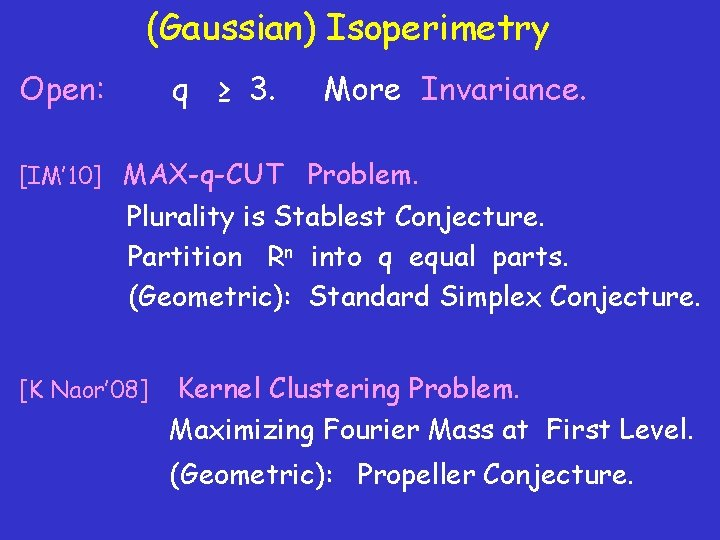(Gaussian) Isoperimetry Open: q ≥ 3. More Invariance. [IM’ 10] MAX-q-CUT Problem. Plurality is Stablest Conjecture. Partition Rn into q equal parts. (Geometric): Standard Simplex Conjecture. [K Naor’ 08] Kernel Clustering Problem. Maximizing Fourier Mass at First Level. (Geometric): Propeller Conjecture.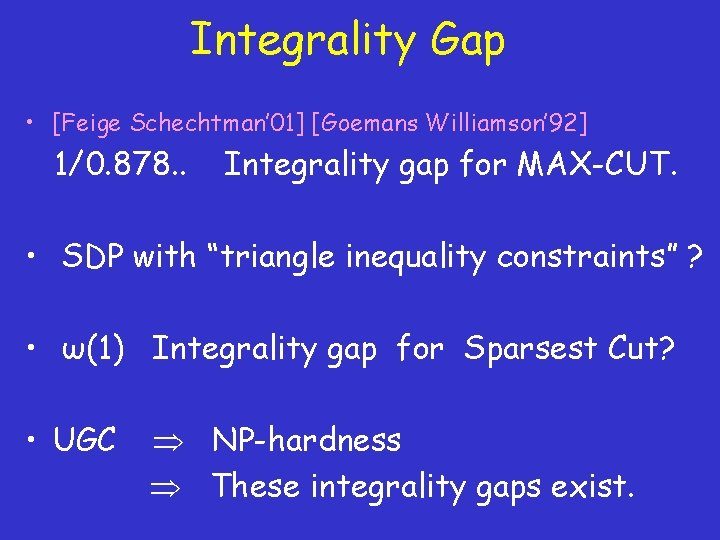Integrality Gap • [Feige Schechtman’ 01] [Goemans Williamson’ 92] 1/0. 878. . Integrality gap for MAX-CUT. • SDP with “triangle inequality constraints” ? • ω(1) Integrality gap for Sparsest Cut? • UGC NP-hardness These integrality gaps exist.[KV’ 05] Integrality Gap for Unique Games SDP v variables v 1 , v 2 , … , vk Orthonormal Bases for Rk k labels u Matchings [k] u 1 , u 2 , … , uk Unique Game G with OPT(G) = o(1) SDP(G) = 1 -o(1)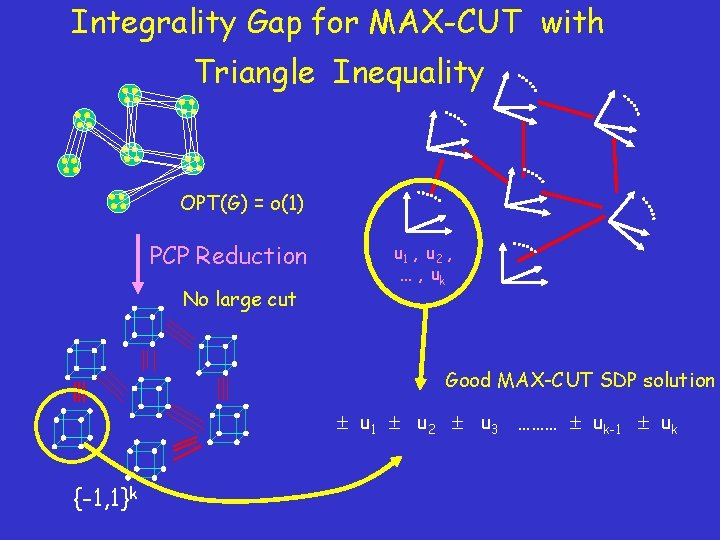Integrality Gap for MAX-CUT with Triangle Inequality OPT(G) = o(1) PCP Reduction No large cut u 1 , u 2 , … , uk Good MAX-CUT SDP solution u 1 u 2 u 3 ……… uk-1 uk {-1, 1}k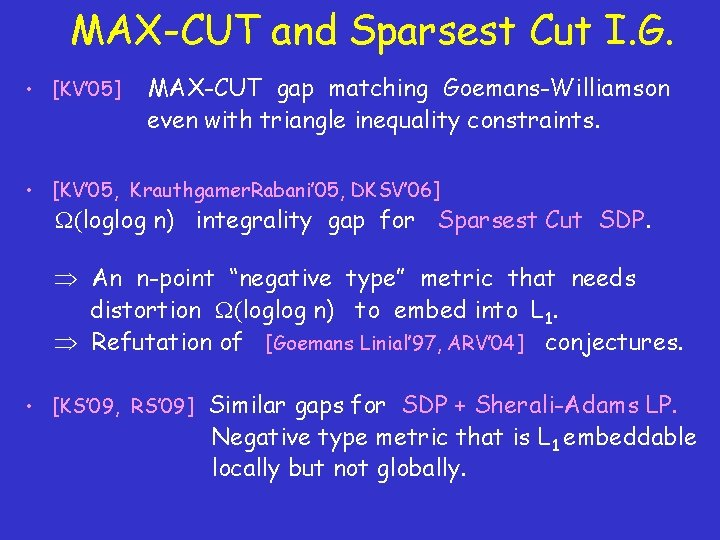MAX-CUT and Sparsest Cut I. G. • [KV’ 05] MAX-CUT gap matching Goemans-Williamson even with triangle inequality constraints. • [KV’ 05, Krauthgamer. Rabani’ 05, DKSV’ 06] (loglog n) integrality gap for Sparsest Cut SDP. An n-point “negative type” metric that needs distortion (loglog n) to embed into L 1. Refutation of [Goemans Linial’ 97, ARV’ 04] conjectures. • [KS’ 09, RS’ 09] Similar gaps for SDP + Sherali-Adams LP. Negative type metric that is L 1 embeddable locally but not globally.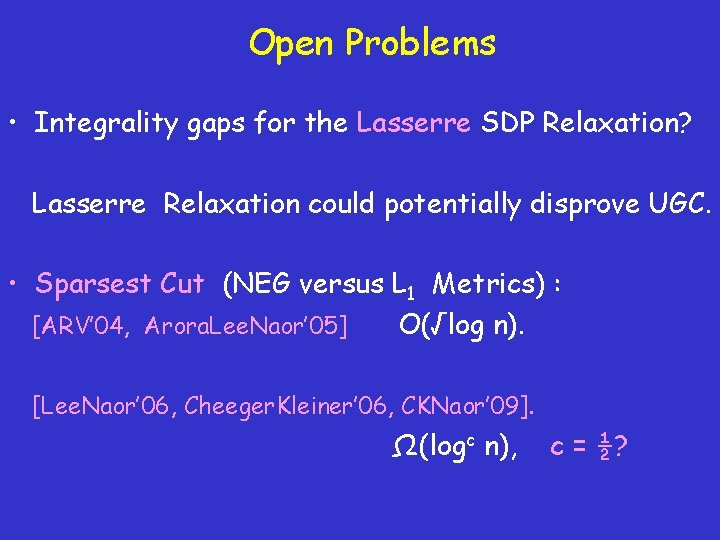Open Problems • Integrality gaps for the Lasserre SDP Relaxation? Lasserre Relaxation could potentially disprove UGC. • Sparsest Cut (NEG versus L 1 Metrics) : [ARV’ 04, Arora. Lee. Naor’ 05] O(√log n). [Lee. Naor’ 06, Cheeger. Kleiner’ 06, CKNaor’ 09]. Ω(logc n), c = ½?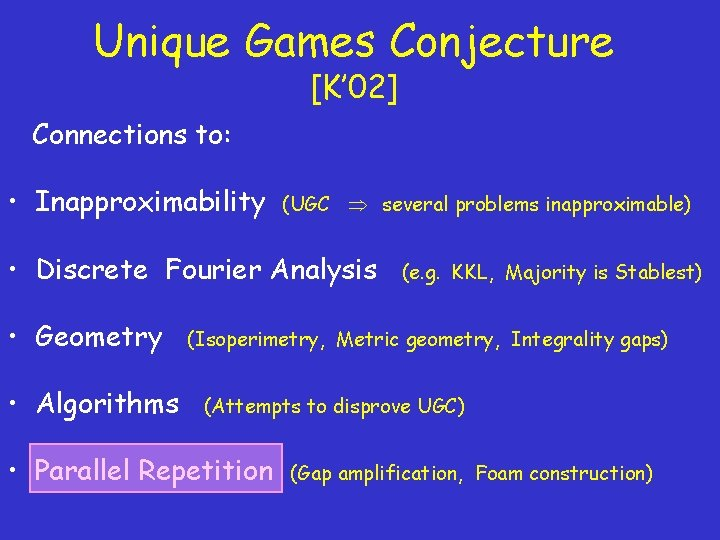Unique Games Conjecture [K’ 02] Connections to: • Inapproximability (UGC several problems inapproximable) • Discrete Fourier Analysis • Geometry • Algorithms (e. g. KKL, Majority is Stablest) (Isoperimetry, Metric geometry, Integrality gaps) (Attempts to disprove UGC) • Parallel Repetition (Gap amplification, Foam construction)Gap Amplification Prove UGC in two steps (? ): • Prove “mild” hardness, i. e. Gap. UG (1 -ε’ , 1 -ε’’ ) is hard. • Amplify gap via parallel repetition to Gap. UG (1 -ε , δ). Note however that even proving “mild” hardness is a huge challenge.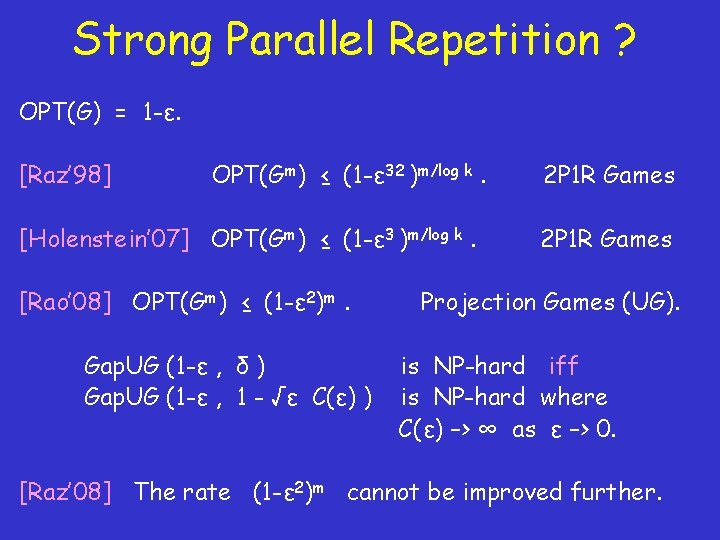Strong Parallel Repetition ? OPT(G) = 1 -ε. [Raz’ 98] OPT(Gm) ≤ (1 -ε 32 )m/log k. [Holenstein’ 07] OPT(Gm) ≤ (1 -ε 3 )m/log k. [Rao’ 08] OPT(Gm) ≤ (1 -ε 2)m. Gap. UG (1 -ε , δ ) Gap. UG (1 -ε , 1 - √ε C(ε) ) 2 P 1 R Games Projection Games (UG). is NP-hard iff is NP-hard where C(ε) –> ∞ as ε –> 0. [Raz’ 08] The rate (1 -ε 2)m cannot be improved further.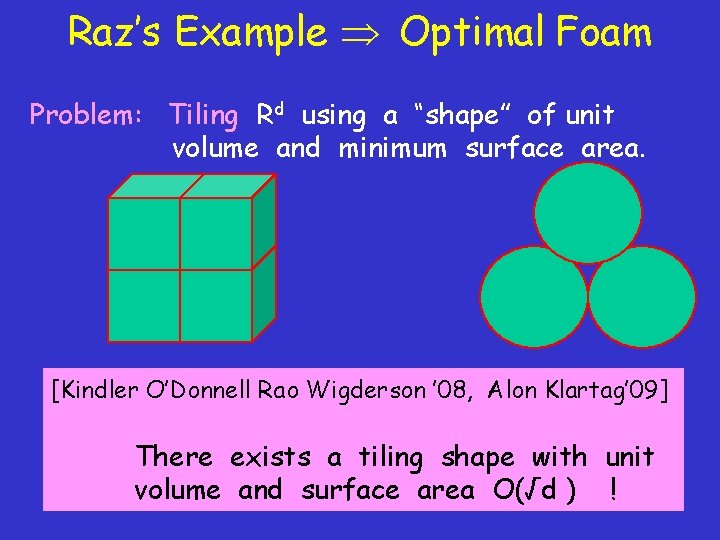Raz’s Example Optimal Foam Problem: Tiling Rd using a “shape” of unit volume and minimum surface area. [Kindler O’Donnell Rao Wigderson ’ 08, Alon Klartag’ 09] Great for tiling: Not good for tiling: There exists a tiling shape with unit Surface area = 2 d Surface area ≈ √d volume and surface area O(√d ) !Conclusion • (Dis)Prove Unique Games Conjecture. • Intermediate between P and NP-complete? • Prove hardness results bypassing UGC. • TSP, Steiner Tree, Scheduling Problems ? • More techniques, connections, results …